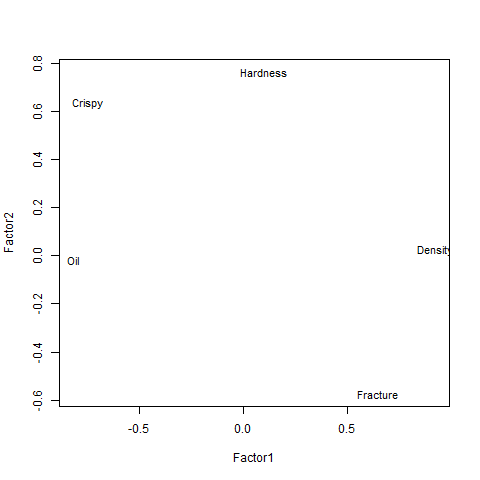GeeksforGeeks App
Open AppBrowser
Continue

# Performing Analysis of a Factor in R Programming – factanal() Function

Factor Analysis also known as Exploratory Factor Analysis is a statistical technique used in R programming to identify the inactive relational structure and further, narrowing down a pool of variables to few variables. The main motive to use this technique is to find out which factor is most responsible for influence in the categorization of weights.

Syntax: factanal(x, factors)

Parameters:
x: represents dataset
factors: specifies number of factors to be fitted

Example:
Let us suppose, there are number of food available in the dataset with their food texture data points such as Oil, Density, Crispy, Fracture, and Hardness.

 `# Reading csv file of food textures``food_textures <``-` `read.csv(``"https://userpage.fu-berlin.de/soga/300/30100_data_sets/food-texture.csv"``)`` ` `food_textures <``-` `food_textures[, ``2``:``6``]`` ` `factor_analysis <``-` `factanal(food_textures, factors ``=` `2``)`` ` `print``(factor_analysis)`` ` `# Output to be present as PNG file ``png(``file` `=` `"factorAnalysisGFG.png"``)`` ` `# Plot factor 1 by factor 2``load <``-` `factor_analysis\$loadings[, ``1``:``2``]`` ` `# Plot graph``plot(load, ``type` `=` `"n"``)``text(load, labels ``=` `names(food_textures), cex ``=` `.``9``)`` ` `# Saving the file``dev.off()`

Output:

```Call:
factanal(x = food_textures, factors = 2)

Uniquenesses:
Oil  Density   Crispy Fracture Hardness
0.334    0.156    0.042    0.256    0.407

Factor1 Factor2
Oil      -0.816
Density   0.919
Crispy   -0.745   0.635
Fracture  0.645  -0.573
Hardness          0.764

Factor1 Factor2